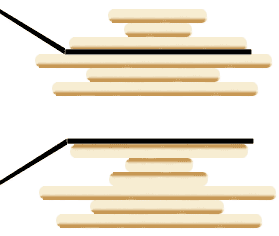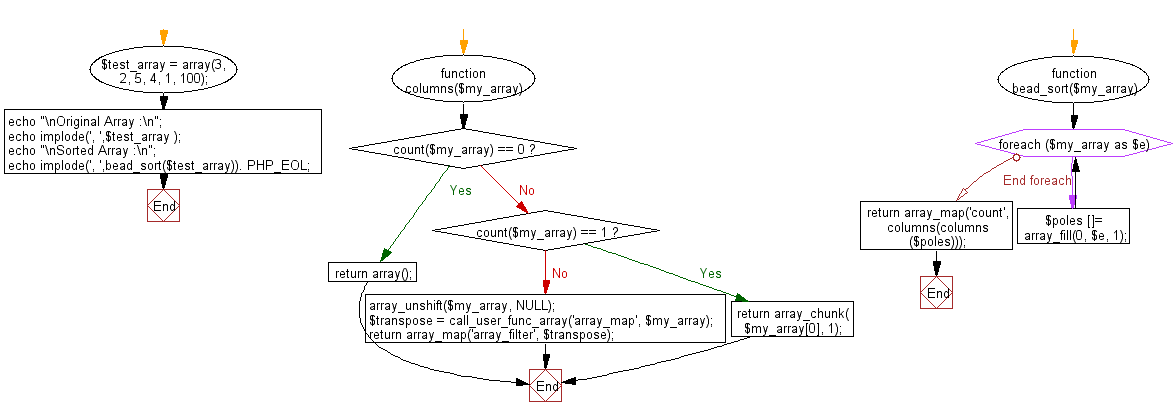# JavaScript Sorting Algorithm: Pancake sort

## JavaScript Sorting Algorithm: Exercise-13 with Solution

Write a JavaScript program to sort a list of elements using Pancake sort.

Pancake sorting is the colloquial term for the mathematical problem of sorting a disordered stack of pancakes in order of size when a spatula can be inserted at any point in the stack and used to flip all pancakes above it. A pancake number is the minimum number of flips required for a given number of pancakes. The problem was first discussed by American geometer Jacob E. Goodman. It is a variation of the sorting problem in which the only allowed operation is to reverse the elements of some prefix of the sequence.

Demonstration of the primary operation :The spatula is flipping over the top three pancakes, with the result seen below. In the burnt pancake problem, their top sides would now be burnt instead of their bottom sides.

Sample Solution : -

HTML Code :

``````<!DOCTYPE html>
<html>
<meta charset="utf-8">
<title>JavaScript program of Pancake sort</title>
<body></body>
</html>
```
```

JavaScript Code :

``````function pancake_sort(arr) {
for (var i = arr.length - 1; i >= 1; i--) {
// find the index of the largest element not yet sorted
var max_idx = 0;
var max = arr;
for (var j = 1; j <= i; j++) {
if (arr[j] > max) {
max = arr[j];
max_idx = j;
}
}

if (max_idx == i)
continue; // element already in place

var new_slice;

// flip arr max element to index 0
if (max_idx > 0) {
new_slice = arr.slice(0, max_idx+1).reverse();
for ( j = 0; j <= max_idx; j++)
arr[j] = new_slice[j];
}

// then flip the max element to its place
new_slice = arr.slice(0, i+1).reverse();
for ( j = 0; j <= i; j++)
arr[j] = new_slice[j];
}
return arr;
}

var arra = [3, 0, 2, 5, -1, 4, 1];
console.log("Original Array Elements");
console.log(arra);
console.log("Sorted Array Elements");
console.log(pancake_sort(arra, 0, 5));
```
```

Sample Output:

```Original Array Elements
[3,0,2,5,-1,4,1]
Sorted Array Elements
[-1,0,1,2,3,4,5]
```

Flowchart :Live Demo :

See the Pen searching-and-sorting-algorithm-exercise-13 by w3resource (@w3resource) on CodePen.

Improve this sample solution and post your code through Disqus

What is the difficulty level of this exercise?

Test your Programming skills with w3resource's quiz.

﻿